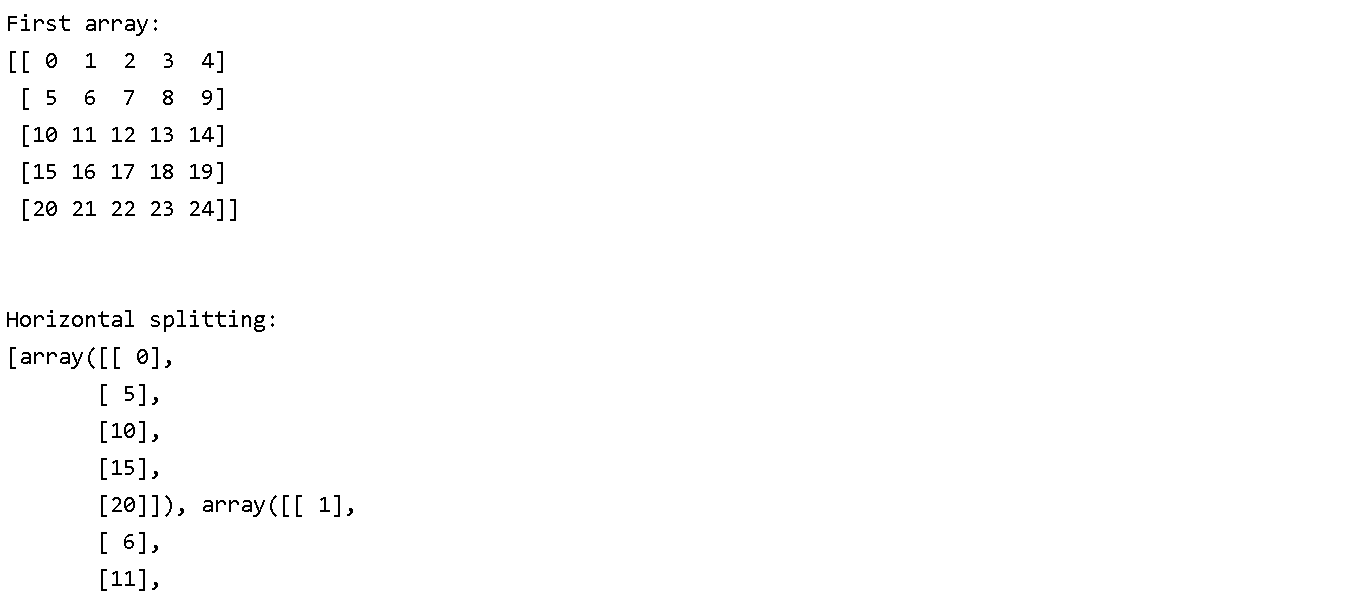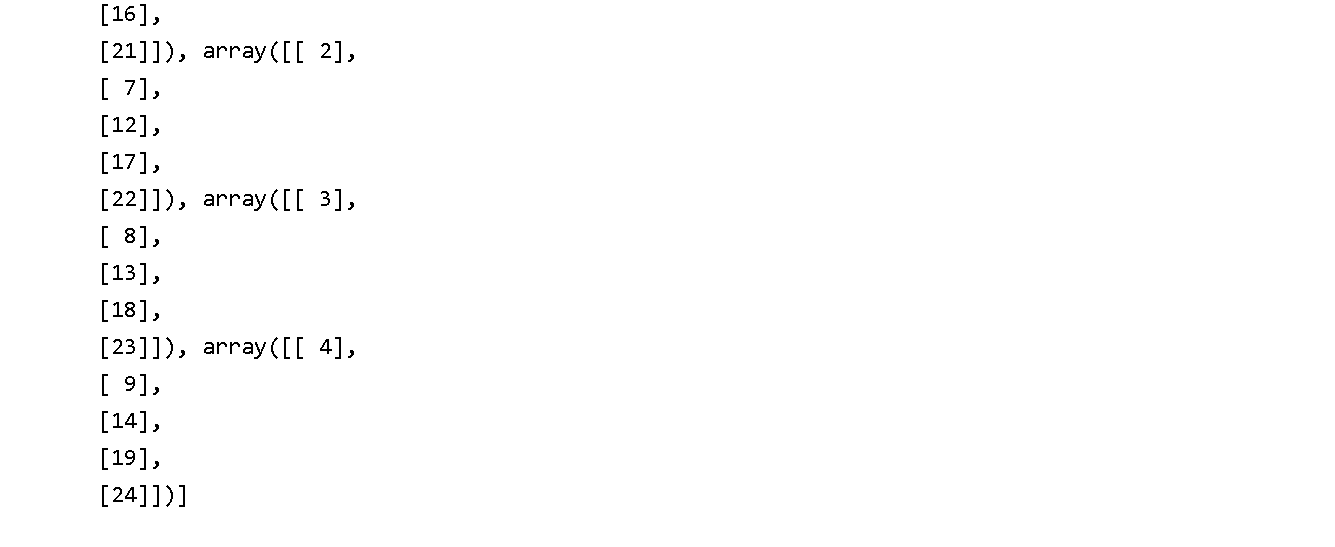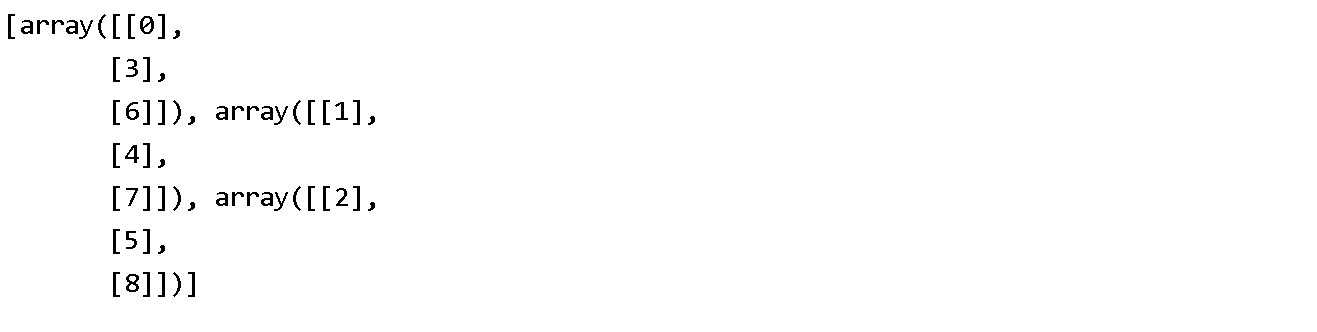### Python Numpy hsplit()

Description
NumPy generally stands for Numerical Python and it is a general purpose array processing package.it always provides a high performance multidimensional array object.Numpy is usually for creating an array of n dimensions.

Method Name :NumPy.hsplit(ary, indices_or_sections)
Description : This function is used to split the multiple sub-array horizontally i.e column wise which is equivalent to split axis-1 which regardless of the dimension of the array. We can also call this function a special case of a split() function of n dimensions.

Parameter Name: ary
Description :ndarray
Input array to be split into sub-arrays.

Parameter Name: indices_or_sections
Description :int or 1-D array
It can be an integer, N, showing the number of equal sized subarrays to be formed from the input array along an axis. If this parameter is a 1-D array of sorted integers, the entries indicate the points at which a new subarray is to be created along the axis.

Return :A list of sub-arrays is showing into ary.

The first step would be to give syntax
command:
numpy.hsplit(ary, indices_or_sections)

Example :
Code :
import numpy as np a = np.arange(25).reshape(5,5) print ('First array:') print (a ) print ('\n' ) print ('Horizontal splitting:') b = np.hsplit(a,5) print (b ) print ('\n')

OutputExample :
Code :
import numpy as np arr = np.arange(9).reshape(3,3) abc = np.hsplit(arr, 3) print (abc)

Output